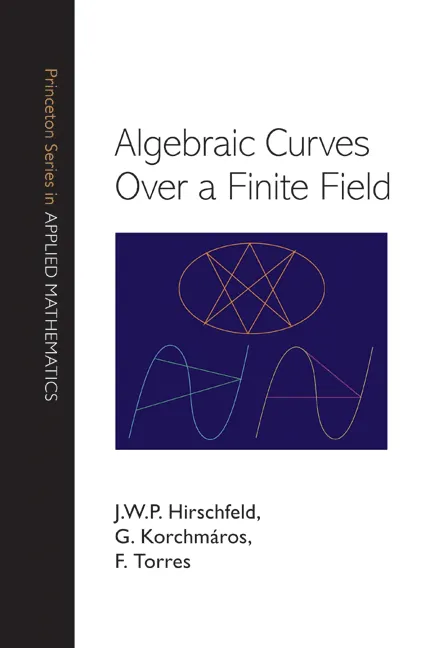#### Algebraic Curves over a Finite Field

J. W. P. Hirschfeld

Audiobooks and ebooks purchased from this site must be accessed on the Princeton University Press app.

# Algebraic Curves over a Finite Field## Hardcover

Price:
\$145.00/£121.00
ISBN:
Published:
Mar 23, 2008
2008
Pages:
744
Size:
6 x 9.25 in.
Illus:
7 line illus. 16 tables.

This book provides an accessible and self-contained introduction to the theory of algebraic curves over a finite field, a subject that has been of fundamental importance to mathematics for many years and that has essential applications in areas such as finite geometry, number theory, error-correcting codes, and cryptology. Unlike other books, this one emphasizes the algebraic geometry rather than the function field approach to algebraic curves.

The authors begin by developing the general theory of curves over any field, highlighting peculiarities occurring for positive characteristic and requiring of the reader only basic knowledge of algebra and geometry. The special properties that a curve over a finite field can have are then discussed. The geometrical theory of linear series is used to find estimates for the number of rational points on a curve, following the theory of Stöhr and Voloch. The approach of Hasse and Weil via zeta functions is explained, and then attention turns to more advanced results: a state-of-the-art introduction to maximal curves over finite fields is provided; a comprehensive account is given of the automorphism group of a curve; and some applications to coding theory and finite geometry are described. The book includes many examples and exercises. It is an indispensable resource for researchers and the ideal textbook for graduate students.